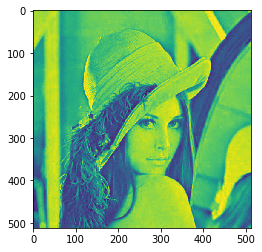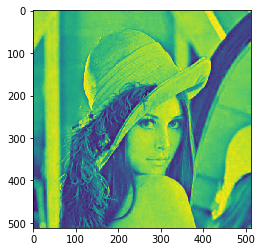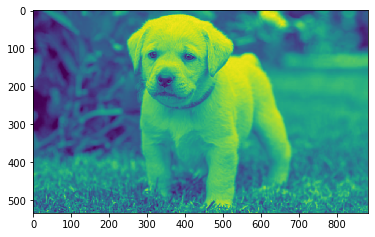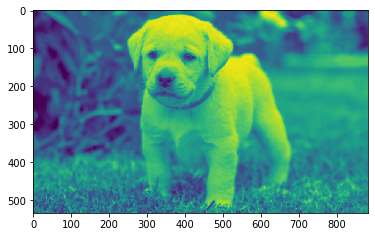# Mahotas – Rank Filter

In this article we will see how we can apply rank filter on image in mahotas. The rank filter, especially filters isolated pixels out, whose intensity differs greatly from that of its immediate neighborhood. Large areas with constant intensity values adjacent to these pixels, as well as edges, are retained after this filter has been used.

In this tutorial we will use “lena” image, below is the command to load it.

`mahotas.demos.load('lena')`

Below is the lena imageIn order to do this we will use ` mahotas.rank_filter` method

Syntax : mahotas.rank_filter(img, neighbour, rank)

Argument : It takes image object and two integer as argument

Return : It returns image object

Note : Input image should be filtered or should be loaded as grey

In order to filter the image we will take the image object which is numpy.ndarray and filter it with the help of indexing, below is the command to do this

`image = image[:, :, 0]`

Below is the implementation

 `# importing required libraries ` `import` `mahotas ` `import` `mahotas.demos ` `from` `pylab ``import` `gray, imshow, show ` `import` `numpy as np ` `import` `matplotlib.pyplot as plt ` `   `  `# loading iamge ` `img ``=` `mahotas.demos.load(``'lena'``) ` `   `  `# filtering image ` `img ``=` `img.``max``(``2``) ` ` `  `print``(``"Image"``) ` `   `  `# showing image ` `imshow(img) ` `show() ` ` `  `# neighbour pixel ` `neighbour ``=` `3` ` `  `# rank ` `rank ``=` `2` ` `  `# applaying rank filter ` `new_img ``=` `mahotas.rank_filter(img, neighbour, rank) ` `  `  ` `  `# showing image ` `print``(``"Rank Filter"``) ` `imshow(new_img) ` `show() `

Output :

`Image``Rank Filter`Another example

 `# importing required libraries ` `import` `mahotas ` `import` `numpy as np ` `from` `pylab ``import` `gray, imshow, show ` `import` `os ` `import` `matplotlib.pyplot as plt ` `  `  `# loading iamge ` `img ``=` `mahotas.imread(``'dog_image.png'``) ` ` `  ` `  `# fltering image ` `img ``=` `img[:, :, ``0``] ` `   `  `print``(``"Image"``) ` `   `  `# showing image ` `imshow(img) ` `show() ` ` `  `# neighbour pixel ` `neighbour ``=` `3` ` `  `# rank ` `rank ``=` `2` ` `  `# applaying rank filter ` `new_img ``=` `mahotas.rank_filter(img, neighbour, rank) ` `  `  ` `  `# showing image ` `print``(``"Rank Filter"``) ` `imshow(new_img) ` `show() `

Output :

`Image``Rank Filter`Attention geek! Strengthen your foundations with the Python Programming Foundation Course and learn the basics.

To begin with, your interview preparations Enhance your Data Structures concepts with the Python DS Course.

My Personal Notes arrow_drop_upCheck out this Author's contributed articles.

If you like GeeksforGeeks and would like to contribute, you can also write an article using contribute.geeksforgeeks.org or mail your article to contribute@geeksforgeeks.org. See your article appearing on the GeeksforGeeks main page and help other Geeks.

Please Improve this article if you find anything incorrect by clicking on the "Improve Article" button below.

Article Tags :

Be the First to upvote.

Please write to us at contribute@geeksforgeeks.org to report any issue with the above content.